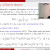Date
May 24 at 16:00 - 18:10, 2021 (JST)
Speaker
Dr. Michiya Mori (Special Postdoctoral Researcher, iTHEMS)
Venue
via Zoom
Language
English

This talk is divided into two independent topics.
In the first part of my talk we consider the order structure of hermitian matrices. Given two matrix domains (open connected sets of n-by-n hermitian matrices), what is the general form of order isomorphisms between them? I will explain that there is a complete correspondence between the class of order isomorphisms and that of biholomorphic mappings.
In the second part we consider the metric structure of the space P(H) of all quantum pure states (= the projective space of a complex Hilbert space H). Wigner's theorem asserts that every surjective isometry of P(H) onto itself is implemented by a unitary or an antiunitary operator. Uhlhorn generalized Wigner's theorem by showing that every bijective transformation of P(H) that preserves orthogonality is implemented by a unitary or an antiunitary operator. We consider some variants of Uhlhorn's result.
The first part is joint work with P. Semrl (Univ. of Ljubljana), and the second part with G.P. Geher (Univ. of Reading). Only basic linear algebra is assumed in both parts.